Question

# For the decomposition of ammonia on a platinum surface at 856 °C 2 NH3-N2 + 3...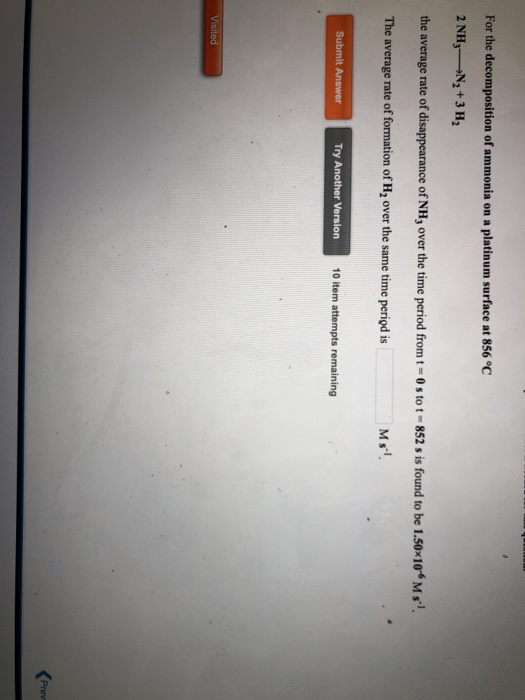For the decomposition of ammonia on a platinum surface at 856 °C 2 NH3-N2 + 3 H2 the average rate of disappearance of NH; over the time period from t = 0 stot - 852 s is found to be 1.50~10 Ms!. The average rate of formation of H, over the same time period is Ms! Submit Answer Try Another Version 10 item attempts remaining Visited Prev

According to the rate kinetics

-1/2 {d[NH3] / dt} = d[N2]/dt = 1/3 {d[H2]/dt} ...................................(negative because of the consumption )

Given that avarage rate of NH3 =1.50*10^-6

Then rate of formation of H2 =

1/2 {d[NH3] / dt} = 1/3 {d[H2]/dt}

=>d[H2]/dt = 3/2  {d[NH3] / dt}

=> d[H2]/dt = 3/2 ( 1.50 * 10 ^-6)

=> d[H2]/dt = 2.25*10^-6 MS^-1

So the rate of formation of H2 = 2.25*10^-6 Ms^-1

Thank you

#### Earn Coins

Coins can be redeemed for fabulous gifts.

Similar Homework Help Questions
• ### For the decomposition of ammonia on a platinum surface at 856 °C 2 NH3-N2 + 3...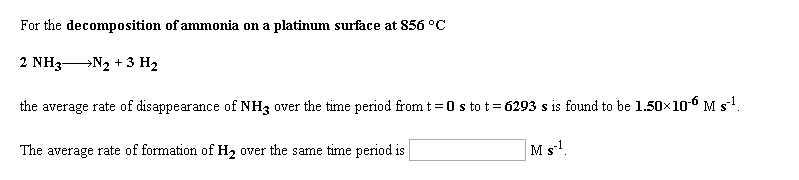For the decomposition of ammonia on a platinum surface at 856 °C 2 NH3-N2 + 3 H2 the average rate of disappearance of NH3 over the time period from t=0 s to t = 6293 s is found to be 1.50X100 Ms? The average rate of formation of H, over the same time period is Ms. For the decomposition of hydrogen peroxide in dilute sodium hydroxide at 20 °C 2 H2O2(aq) +2 H2O(1) + O2(g) the average rate of disappearance...

• ### The decomposition of ammonia on a platinum surface at 856 °C NH3-------> 1/2 N2 + 3/2...

The decomposition of ammonia on a platinum surface at 856 °C NH3-------> 1/2 N2 + 3/2 H2 is zero order in NH3 with a rate constant of 1.50×10-6 M s-1. If the initial concentration of NH3 is 1.00×10-2 M, the concentration of NH3 will be _______________ M after 5.23×103 seconds have passed.

• ### In a study of the decomposition of ammonia on a platinum surface at 856 °C NH31/2...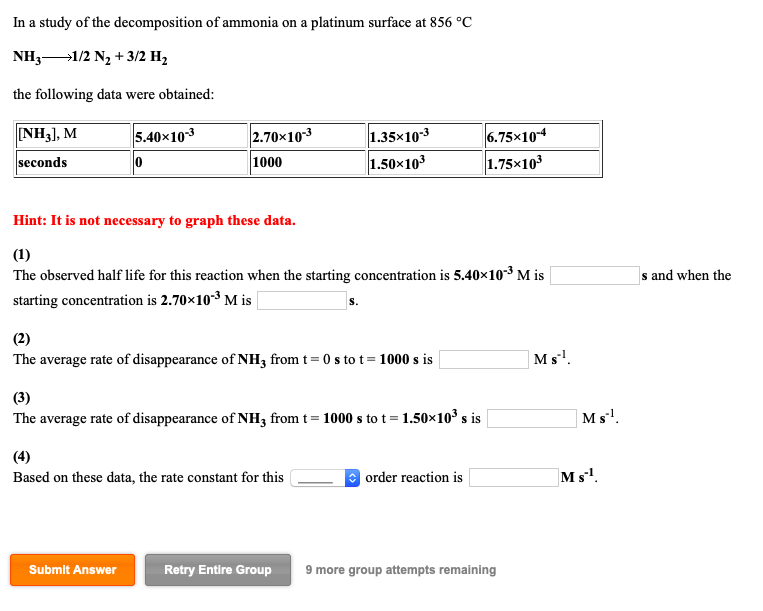In a study of the decomposition of ammonia on a platinum surface at 856 °C NH31/2 N2+3/2 H2 the following data were obtained NH3], M 1.35x10-3 5.40x103 2.70x103 6.75x104 1.50x103 1.75x103 seconds 1000 Hint: It is not necessary to graph these data. (1) The observed half life for this reaction when the starting concentration is 5.40x103 M is s and when the starting concentration is 2.70x103 M is (2) Ms The average rate of disappearance of NH3 from t 0s...

• ### The decomposition of ammonia on a platinum surface at 856 °C NH31/2 N2 + 3/2 H2...

The decomposition of ammonia on a platinum surface at 856 °C NH31/2 N2 + 3/2 H2 is zero order in NH3 with a rate constant of 1.50×10-6 M s-1. If the initial concentration of NH3 is 7.92×10-3 M, the concentration of NH3 will be M after 4.29×103 seconds have passed.

• ### For the gas phase decomposition of dinitrogen pentoxide at 335 K 2 N205 4 NO2 +...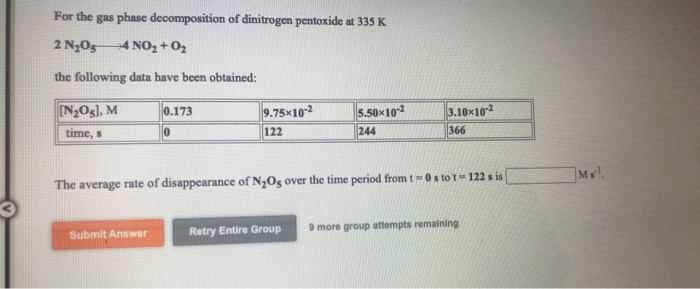For the gas phase decomposition of dinitrogen pentoxide at 335 K 2 N205 4 NO2 + O2 the following data have been obtained: 0.173 [N2O3], M time, s 9.75x10-2 122 5.50x10-2 244 3.10x102 366 Ms! The average rate of disappearance of N,Os over the time period from t=0 stot - 122 sis Submit Answer Retry Entire Group 9 more group attempts remaining For the gas phase decomposition of hydrogen iodide at 700 K 2 HI -H2 +12 the following data...

• ### Question 7 A catalyst ) 2 NH3 ( g N2 (9) + 3 H2 ( g...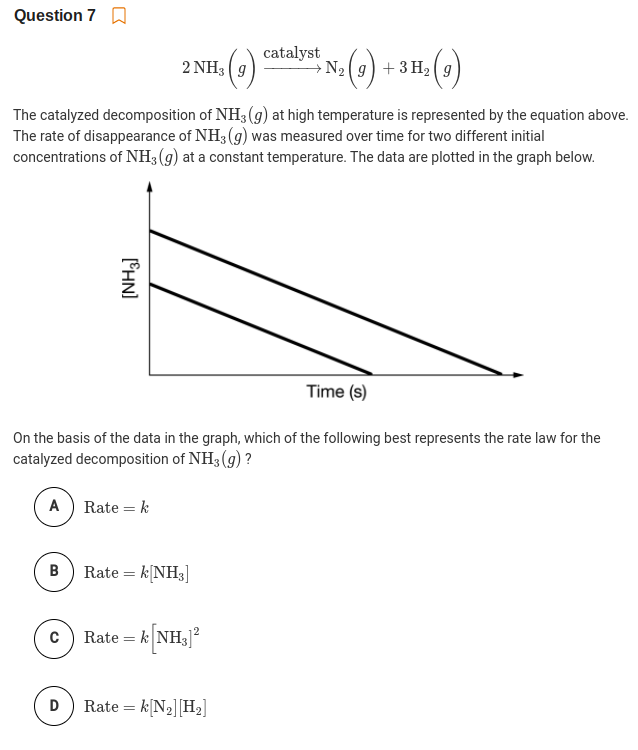Question 7 A catalyst ) 2 NH3 ( g N2 (9) + 3 H2 ( g The catalyzed decomposition of NH3 (g) at high temperature is represented by the equation above. The rate of disappearance of NH3 (g) was measured over time for two different initial concentrations of NH3 (g) at a constant temperature. The data are plotted in the graph below. Time (s) On the basis of the data in the graph, which of the following best represents the...

• ### In a study of the decomposition of ammonia on a tungsten surface at 1100 °C NH31/2...

In a study of the decomposition of ammonia on a tungsten surface at 1100 °C NH31/2 N2 + 3/2 H2 the following data were obtained: [NH3], M 6.69×10-3 3.35×10-3 1.68×10-3 8.40×10-4 seconds 0 441 661 772 Hint: It is not necessary to graph these data. (1) The observed half life for this reaction when the starting concentration is 6.69×10-3 M is s and when the starting concentration is 3.35×10-3 M is s. (2) The average rate of disappearance of NH3...

• ### Use the Referraces to access important values if needed for this question In a study of...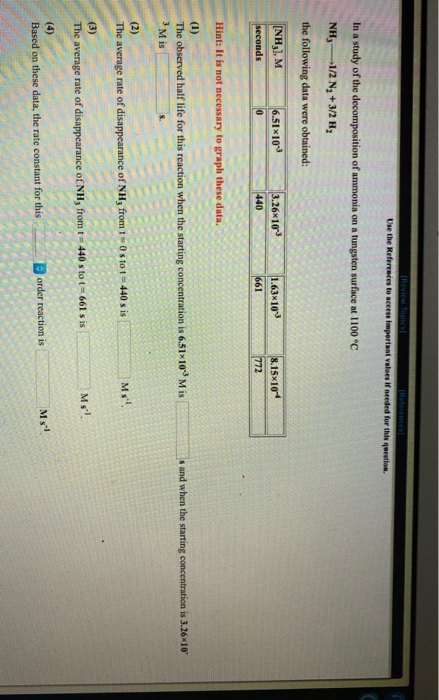Use the Referraces to access important values if needed for this question In a study of the decomposition of ammonia on a tungsten surface at 1100 °C NH3 1/2 N2 + 3/2 H, the following data were obtained: (NH3), M seconds 6.51x101 0 3 .26x1019 440 163x108 8.15x10 Hint: It is not necessary to graph these data. The observed half life for this reaction when the star s and when the starting concentration is 3.26x10 M is S. (2) The...

• ### The decomposition of ammonia gas is endothermic, AH = 92 kJ/mol. 2 NH3() = N2(g) +...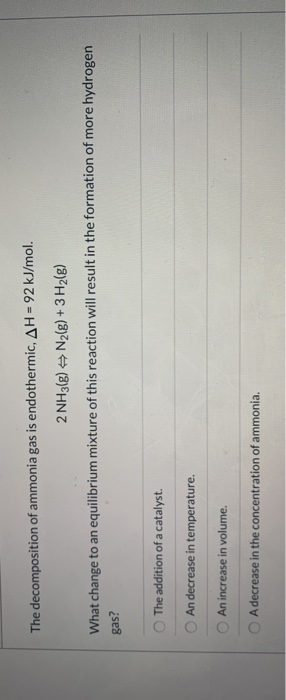The decomposition of ammonia gas is endothermic, AH = 92 kJ/mol. 2 NH3() = N2(g) + 3H2(g) What change to an equilibrium mixture of this reaction will result in the formation of more hydrogen gas? The addition of a catalyst. An decrease in temperature. An increase in volume. A decrease in the concentration of ammonia.

• ### For the reaction 2 NH3 --> 3 H2 + 2 N2 The rate of disappearance of...

For the reaction 2 NH3 --> 3 H2 + 2 N2 The rate of disappearance of ammonia is -0.00698 M/hr Calculate the rate of appearance of hydrogen gas Calculate the rate of appearance of nitrogen gas